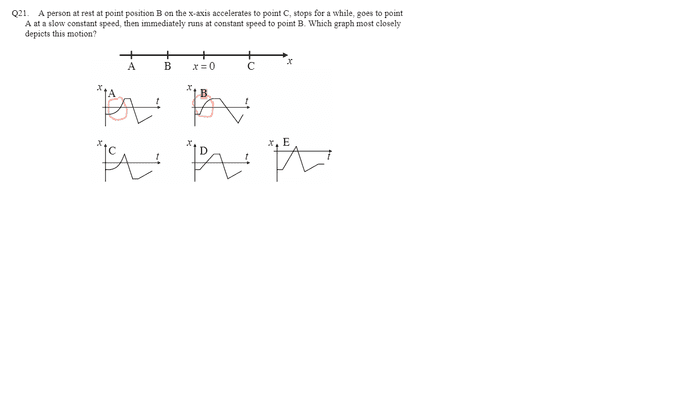# Curves on a Position time graph

• qwertyqwert321

## Homework Statement## The Attempt at a Solution

I knew the answer was between A and B, but the inward curves and outward curves on the position time graph confused me , what exactly does it mean?
-does A have an increasing velocity and then B has a decreasing velocity? I am really confused with the slopes

does A have an increasing velocity and then B has a decreasing velocity?
Yes.

I don't think any of the graphs are correct. The one that is the closest is still wrong because the absolute value of the gradient of the segment that is described as 'slow constant speed' is greater than that for the segment described as 'running'. They should replace 'runs' by 'strolls' or even 'crawls'.

I think the correct answer would have the first two segments of A followed by the last two segments of B.

The answer is probably B. The problem statement doesn't state the acceleration is constant, so an initially higher amount of acceleration that reduces as the constant speed is approached would be allowed, so B's curve in position versus time during acceleration isn't an issue. (Consider the case of a sprinter taking off from blocks). This leaves the slower constant negative velocity followed by faster positive velocity to decide between A and B.

•andrewkirk
The problem statement doesn't state the acceleration is constant
Great lateral thinking!